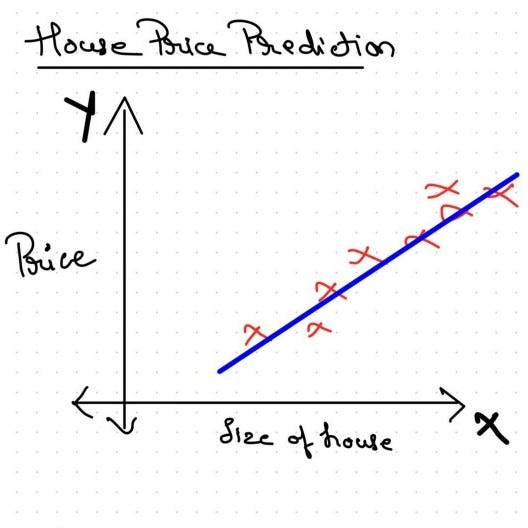# Deep Learning Specialization Part-one

Source: Deep Learning on Medium# Introduction to Deep Learning

## What is Neural Network ?

Basic intuition of neural network using house price prediction as toy example.

Problem statement : Predict price of a house by looking at data describing the house.

Technically, if X is the input data we have about the house and Y is price of that house, we want to find a mapping between X to Y.

In order to look at how neural network solve this problem we need to start from the most simplest neural network, a Neuron. Neurons are fundamental building of any neural network and they map their input to output via some function mapping.

A typical mapping function for a neuron looks like taking summation of all the inputs multiplied by there weights and applying a activation (non-linear) function to that value to give out final value as output.